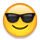Multi Formula/Sheet Help

08/18/18 Edited 12/09/19

Hi

Been struggling with this for ages, I can get the two elements of this formula to work separately but not together - I hope the below makes sense!I am trying to get a formula to:

A) Search a column on another sheet to see if a reference number is present

The below works but gives me the total count

=COUNTIF({SHEET1}, REFERENCE1)Return a result based on if a tick box is unchecked for the above confirmed reference

There would only be one unchecked entry on the other sheet of the reference number and loads with it checked, I only need to confirm if the reference is present and the tickbox is unchecked on that line.

Hope you can help, thanks

• How would you want your result displayed?

thinkspi.com

• Just a boolean response for if the box is ticked or not good, generally the reference would always be present.

Thanks for help Paul

• You're on the right track. Give this a try...

=IF(COUNTIFS({Reference Sheet Rang 1}, [Column Name]@row, {Reference Sheet Range 2}, 0) = 1, 1)

{Reference Sheet Range 1}: The column on your reference sheet containing the list of numbers.

[Column Name]@row: Simply change "Column Name" to the appropriate column name containing the number on your target sheet (where you're putting the formula).

{Reference Sheet Range 2}: The column on your reference sheet containing the checkboxes.

What this does is it will look on your reference sheet for the value that is in the same row as your formula. It will then count how many time it finds an unchecked box next to that number on your reference sheet. If that count is 1, then it will check the box where you have your formula. Otherwise it will remain unchecked letting you know that either no instances or more than one instance was found (which I assume are both issues you want to address by the sound of your post).

thinkspi.com